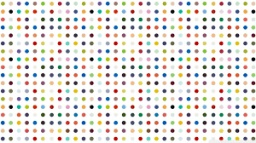# Circumference 6382

Jan bought a large rectangle plot with a circumference of 90 meters. He divided it into three rectangular plots. The shorter side has all three plots of the same length. Their longer sides are three consecutive natural numbers. Find out each plot's dimensions and the whole plot's area. Find all the solutions!

a1 =  3 m
a2 =  6 m
a3 =  9 m
S3 =  324 m2

### Step-by-step explanation:Did you find an error or inaccuracy? Feel free to write us. Thank you!

Tips for related online calculators
Do you solve Diofant problems and looking for a calculator of Diofant integer equations?
Do you have a linear equation or system of equations and looking for its solution? Or do you have a quadratic equation?

#### You need to know the following knowledge to solve this word math problem:

We encourage you to watch this tutorial video on this math problem: